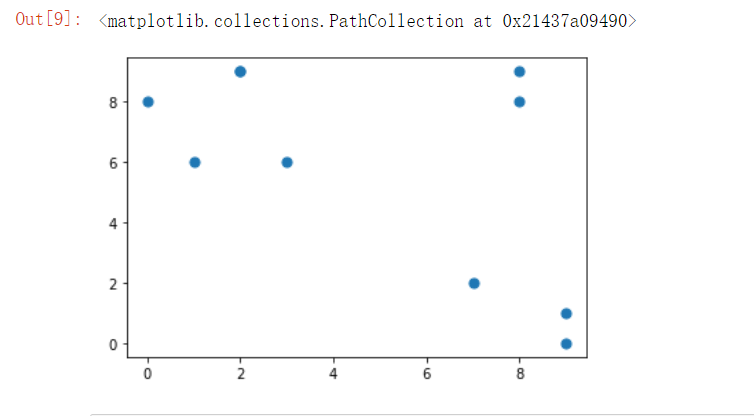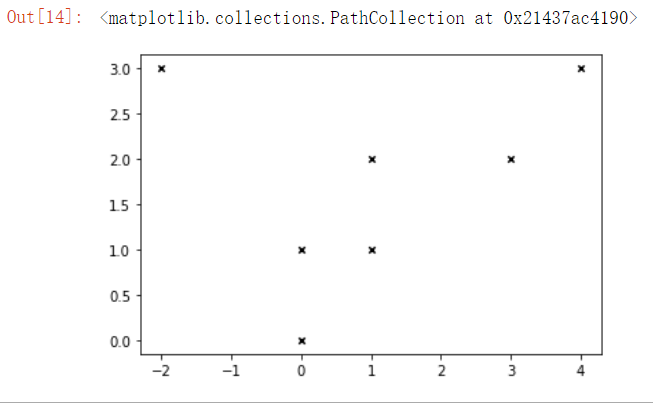# python—-scatter绘画散点图

import matplotlib.pyplot  as plt

plt.scatter(x,y,

s = 20

c='b'

marker=‘o’

cmap=None,

norm=None,

vmin=None,

vmax=None,

alpha=None,

linewidths=None,

)

 x,y 散点图的数据点 形如shape(n,)数组 s 标量或 形如shape(n,)数组，可选，默认20 c 表示色彩或颜色序列，默认蓝色‘b’。注意c不应当是单一的RGB数字或者RGBA序列，c可以是一个RGB或者RGBA二维行数组 marker MarkerStyle，表示的是标记的样式，可选，默认’o’。 cmap Colormap，标量或者是一个colormap的名字，cmap仅仅当c是一个浮点数数组的时候才使用。如果没有申明就是image.cmap，可选，默认None。 norm Normalize，数据亮度在0-1之间，也是只有c是一个浮点数的数组的时候才使用。如果没有申明，就是默认None。 vmin，vmax 标量，当norm存在的时候忽略。用来进行亮度数据的归一化，可选，默认None。 alpha 标量，0-1之间，可选，默认None。 linewidths 也就是标记点的长度，默认None。

``````import numpy as np
import pandas as pd
import matplotlib.pyplot as plt

x=np.random.randint(0,10,10)
y=np.random.randint(0,10,10)
print(x)
print(y)
plt.scatter(x,y,s=50)默认形状圆点，颜色b 蓝色，大小50
``````

out:
[5 1 5 3 1 5 0 5 4 7]
[8 2 7 7 8 6 3 8 5 9]``````import numpy as np
import pandas as pd
import matplotlib.pyplot as plt
x = np.array([[0,0],[0,1],[1,1],[3,2],[-2,3],[1,2],[4,3]])
print(x)
plt.scatter(x[:,0],x[:,1],marker='x',color='k',s=20)#点的形状x，颜色黑色，大小20``````

out:

```[[ 0  0]
[ 0  1]
[ 1  1]
[ 3  2]
[-2  3]
[ 1  2]
[ 4  3]]```